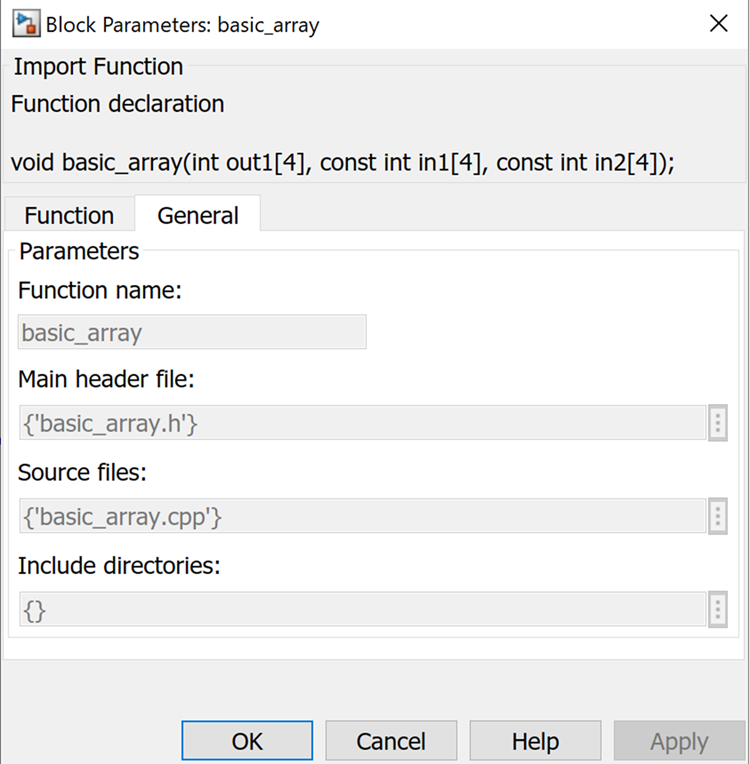# Step 1: Set up the Import Function Example - 2020.2 English

Document ID
UG1498
Release Date
2021-01-22
Version
2020.2 English
In the MATLAB Current Folder panel, navigate to Lab2\Section1 folder.
1. Double-click the basic_array.cpp and basic_array.h files to view the source code in the MATLAB Editor.

These are the source files for a simple `basic_array` function in `C++`, which calculates the sum of two arrays of size 4. You will import this function as a Model Composer block using the `xmcImportFunction` function.

The input and output ports for the generated block are determined by the signature of the source function. Model Composer identifies arguments specified with the `const` qualifier as inputs to the block, and all other arguments as outputs.

Note: For more details and other options for specifying the direction of the arguments, see the Model Composer and System Generator User Guide (UG1483).
Important: You can use the `const` qualifier in the function signature to identify the inputs to the block or use the pragma `INPORT`.

In the case of the `basic_array` function, the `in1` and `in2` arguments are identified as inputs.

``````void basic_array(
uint8_t out1,
const uint8_t in1,
const uint8_t in2)
``````
2. To learn how to use the `xmcImportFunction` function, type `help xmcImportFunction` at the MATLAB command prompt to view the help text and understand the function signature.
3. Open the `import_function.m` MATLAB script, and fill in the required fields for the `xmcImportFunction` function in this way:
``xmcImportFunction('basic_array_library', {'basic_array'}, 'basic_array.h', {'basic_array.cpp'}, {});``

The information is defined as follows:

Library Name
`basic_array_library`. This is the name of the Simulink library that is created with the new block.
Function Names
`basic_array`. This is the name of the function that you want to import as a block.
`basic_array.h`. This is the header file for the function.
Source Files
`basic_array.cpp`. This is the source file for the imported function.
Search Paths
This argument is used to specify the search path(s) for header files. In this example, there are no additional search paths to specify and hence you can leave it as { } which indicates none.
Note: Look at import_function_solution.m in the solution folder for the completed version.
4. Run the `import_function.m` script from the MATLAB command line:
``>>run('import_function.m')``

Notice that a Simulink library model opens up with the generated block `basic_array`.